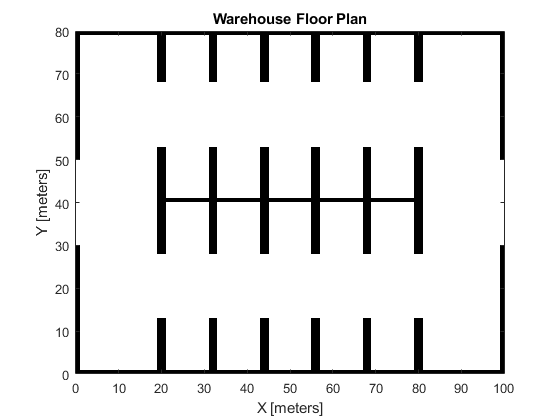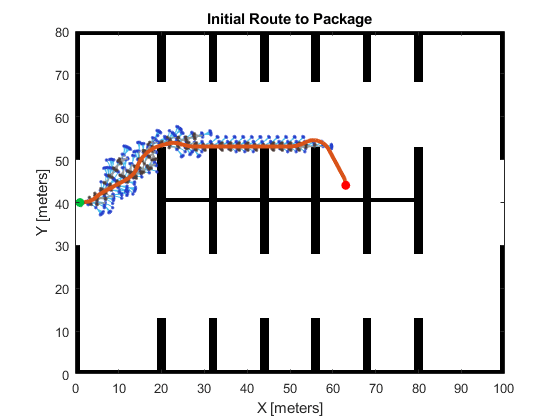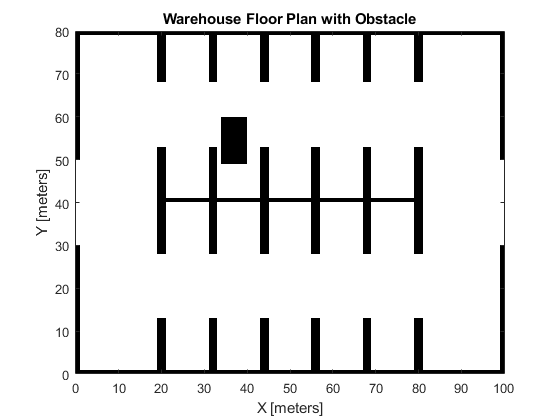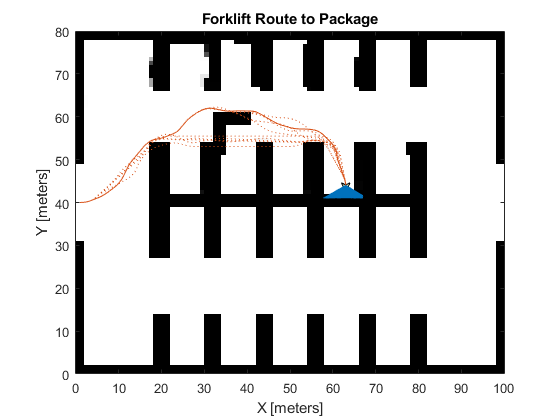# Dynamic Replanning on an Indoor Map

This example shows how to perform dynamic replanning on a warehouse map with a range finder and an A* path planner.

### Create Warehouse Map

Define a `binaryOccupancyMap` that contains the floor plan for the warehouse. This information will be used to create an initial route from the warehouse entrance to the package pickup.

```map = binaryOccupancyMap(100, 80, 1); occ = zeros(80, 100); occ(1,:) = 1; occ(end,:) = 1; occ([1:30, 51:80],1) = 1; occ([1:30, 51:80],end) = 1; occ(40,20:80) = 1; occ(28:52,[20:21 32:33 44:45 56:57 68:69 80:81]) = 1; occ(1:12, [20:21 32:33 44:45 56:57 68:69 80:81]) = 1; occ(end-12:end, [20:21 32:33 44:45 56:57 68:69 80:81]) = 1; setOccupancy(map, occ) figure show(map) title('Warehouse Floor Plan')```### Plan Route to Pickup

Create a `plannerHybridAStar` and use the previously created floor plan to generate an initial route.

```estMap = occupancyMap(occupancyMatrix(map)); vMap = validatorOccupancyMap; vMap.Map = estMap; planner = plannerHybridAStar(vMap, 'MinTurningRadius', 2); entrance = [1 40 0]; packagePickupLocation = [63 44 -pi/2]; route = plan(planner, entrance, packagePickupLocation); route = route.States; % Get poses from the route. rsConn = reedsSheppConnection('MinTurningRadius', planner.MinTurningRadius); startPoses = route(1:end-1,:); endPoses = route(2:end,:); rsPathSegs = connect(rsConn, startPoses, endPoses); poses = []; for i = 1:numel(rsPathSegs) lengths = 0:0.1:rsPathSegs{i}.Length; [pose, ~] = interpolate(rsPathSegs{i}, lengths); poses = [poses; pose]; end figure show(planner) title('Initial Route to Package')```### Place an Obstacle in the Route

Add an obstacle to the map that is on the route the forklift will take to the package.

```obstacleWidth = 6; obstacleHeight = 11; obstacleBottomLeftLocation = [34 49]; values = ones(obstacleHeight, obstacleWidth); setOccupancy(map, obstacleBottomLeftLocation, values) figure show(map) title('Warehouse Floor Plan with Obstacle')```### Specify the Range Finder

Create the range finder using the `rangeSensor` object.

```rangefinder = rangeSensor('HorizontalAngle', pi/2); numReadings = rangefinder.NumReadings;```

### Update the Route Based on Range Finder Readings

Advance the forklift forward using the poses from the path planner. Get the new obstacle detections from the range finder and insert them into the estimated map. If the route is now occupied in the updated map, recalculate the route. Repeat until the goal is reached.

```% Setup visualization. helperViz = HelperUtils; figure show(estMap) hold on robotPatch = helperViz.plotRobot(gca, poses(1,:)); rangesLine = helperViz.plotScan(gca, poses(1,:), ... NaN(numReadings,1), ones(numReadings,1)); axesColors = get(gca, 'ColorOrder'); routeLine = helperViz.plotRoute(gca, route, axesColors(2,:)); traveledLine = plot(gca, NaN, NaN); title('Forklift Route to Package') hold off idx = 1; tic; while idx <= size(poses,1) % Insert range finder readings into map. [ranges, angles] = rangefinder(poses(idx,:), map); insertRay(estMap, poses(idx,:), ranges, angles, ... rangefinder.Range(end)); % Update visualization. show(estMap, 'FastUpdate', true); helperViz.updateWorldMap(robotPatch, rangesLine, traveledLine, ... poses(idx,:), ranges, angles) drawnow % Regenerate route when obstacles are detected in the current one. isRouteOccupied = any(checkOccupancy(estMap, poses(:,1:2))); if isRouteOccupied && (toc > 0.5) % Calculate new route. planner.StateValidator.Map = estMap; route = plan(planner, poses(idx,:), packagePickupLocation); route = route.States; % Get poses from the route. startPoses = route(1:end-1,:); endPoses = route(2:end,:); rsPathSegs = connect(rsConn, startPoses, endPoses); poses = []; for i = 1:numel(rsPathSegs) lengths = 0:0.1:rsPathSegs{i}.Length; [pose, ~] = interpolate(rsPathSegs{i}, lengths); poses = [poses; pose]; %#ok<AGROW> end routeLine = helperViz.updateRoute(routeLine, route); idx = 1; tic; else idx = idx + 1; end end```